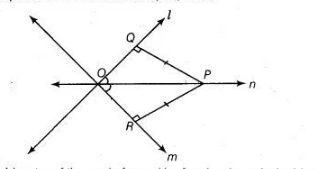# Two lines I and m intersect at the`
Question:

Two lines $I$ and $m$ intersect at the point 0 and $P$ is a point on a line $n$ passing through the point 0 such that $P$ is equidistant from $I$ and $m$. Prove that $n$ is the bisector of the angle formed by $I$ and $m$.

Solution:

Given Two lines $l$ and $m$ intersect at the point $O$ and $P$ is a point on a line $n$ passing through O such that $P$ is equidistant from $l$ and $m$. i.e., $P Q=P R$.To prove $n$ is the bisector of the angle formed by $l$ and $m$ i.e., $n$ is the bisector of $\angle Q O R$. Proof in $\triangle O Q P$ and $\triangle O R P$,

$\angle P Q O=\angle P R O=90^{\circ}$

[since, $P$ in equidistant from $l$ and $m$, so $P Q$ and $P R$ should be perpendicular to lines $l$ and $m$ respectively]

$O P=O P \quad$ [common side]

$P Q=P R$ [given]

$\therefore$ $\triangle O Q P \cong \triangle O R P$ [by RHS congruence rule]

$\Rightarrow$ $\angle P O Q=\angle P O R$ [by CPCT]

Hence, $n$ is the bisector of $\angle Q O R$.

Hence proved.